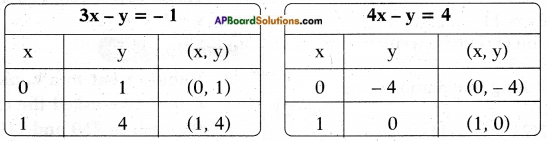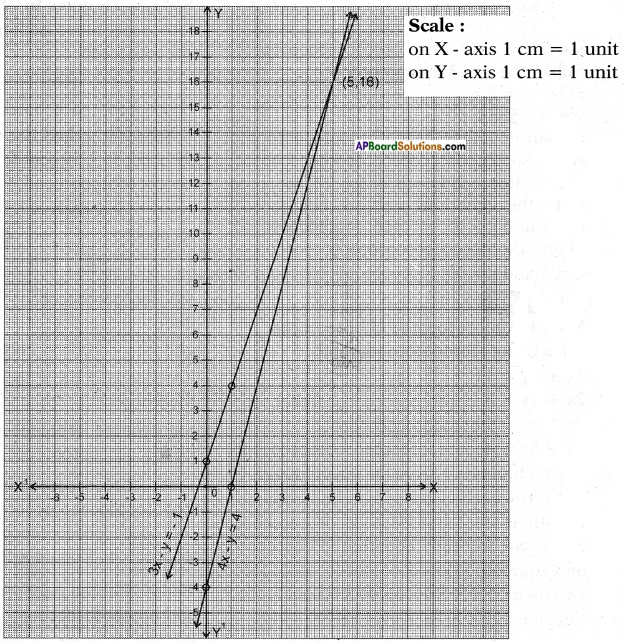# AP SSC 10th Class Maths Solutions Chapter 4 Pair of Linear Equations in Two Variables Ex 4.1

AP State Board Syllabus AP SSC 10th Class Maths Textbook Solutions Chapter 4 Pair of Linear Equations in Two Variables Ex 4.1 Textbook Questions and Answers.

## AP State Syllabus SSC 10th Class Maths Solutions 4th Lesson Pair of Linear Equations in Two Variables Exercise 4.1

### 10th Class Maths 4th Lesson Pair of Linear Equations in Two Variables Ex 4.1 Textbook Questions and Answers

Question 1.
By comparing the ratios $$\frac{a_{1}}{a_{2}}$$, $$\frac{b_{1}}{b_{2}}$$, $$\frac{c_{1}}{c_{2}}$$ K find out whether the lines represented by the following pairs of linear equations intersect at a point, are parallel or are coincident.
a) 5x – 4y + 8 = 0
7x + 6y – 9 = 0
Given: 5x – 4y + 8 = 0
7x + 6y – 9 = 0
$$\frac{a_{1}}{a_{2}}$$ = $$\frac{5}{7}$$; $$\frac{b_{1}}{b_{2}}$$ = $$\frac{-4}{6}$$; $$\frac{c_{1}}{c_{2}}$$ = $$\frac{8}{-9}$$
∴ $$\frac{a_{1}}{a_{2}}$$ ≠ $$\frac{b_{1}}{b_{2}}$$
Hence the given pair of linear equations represents a pair of intersecting lines.

b) 9x + 3y + 12 = 0
18x + 6y + 24 = 0
Given : 9x + 3y + 12 = 0
18x + 6y + 24= 0
$$\frac{a_{1}}{a_{2}}$$ = $$\frac{9}{18}$$ = $$\frac{1}{2}$$;
$$\frac{b_{1}}{b_{2}}$$ = $$\frac{3}{6}$$ = $$\frac{1}{2}$$;
$$\frac{c_{1}}{c_{2}}$$ = $$\frac{12}{24}$$ = $$\frac{1}{2}$$
∴ $$\frac{a_{1}}{a_{2}}$$ = $$\frac{b_{1}}{b_{2}}$$ = $$\frac{c_{1}}{c_{2}}$$
The lines are coincident.c) 6x – 3y + 10 = 0
2x – y + 9 = 0
Given: 6x – 3y + 10 = 0
2x – y + 9 = 0
$$\frac{a_{1}}{a_{2}}$$ = $$\frac{6}{2}$$ = $$\frac{3}{1}$$;
$$\frac{b_{1}}{b_{2}}$$ = $$\frac{-3}{-1}$$ = $$\frac{3}{1}$$;
$$\frac{c_{1}}{c_{2}}$$ = $$\frac{10}{9}$$
Here $$\frac{a_{1}}{a_{2}}$$ = $$\frac{b_{1}}{b_{2}}$$ ≠ $$\frac{c_{1}}{c_{2}}$$
∴ The lines are parallel.

Question 2.
Check whether the following equations are consistent or inconsistent. Solve them graphically. (AS2, AS5)
a) 3x + 2y = 8
2x – 3y = 1
Given equaions are 3x + 2y = 8 and 2x – 3y = 1
$$\frac{a_{1}}{a_{2}}$$ = $$\frac{3}{2}$$;
$$\frac{b_{2}}{b_{-3}}$$ = $$\frac{-4}{6}$$;
$$\frac{a_{1}}{a_{2}}$$ ≠ $$\frac{b_{1}}{b_{2}}$$
Hence the linear equations are consistent.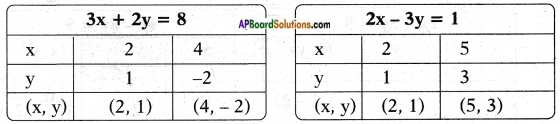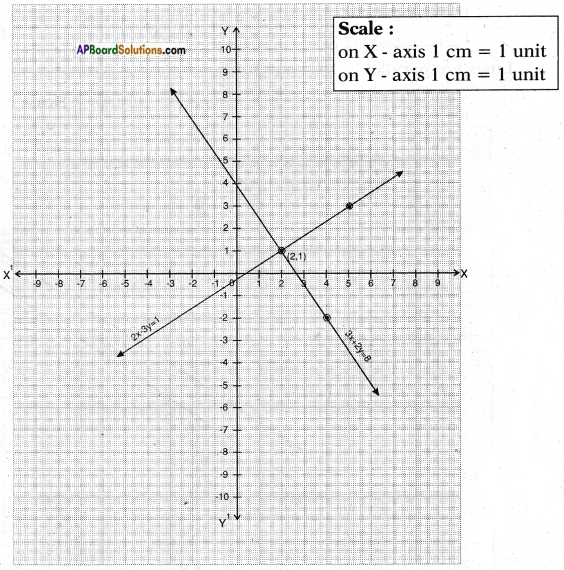The lines intersect at (2, 1), so the solution is (2, 1).

b) 2x – 3y = 8
4x – 6y = 9
Given: 2x – 3y = 8 and 4x – 6y = 9
$$\frac{a_{1}}{a_{2}}$$ = $$\frac{2}{4}$$ = $$\frac{1}{2}$$;
$$\frac{b_{1}}{b_{2}}$$ = $$\frac{-3}{-6}$$ = $$\frac{1}{2}$$;
$$\frac{c_{1}}{c_{2}}$$ = $$\frac{8}{9}$$
∴ $$\frac{a_{1}}{a_{2}}$$ = $$\frac{b_{1}}{b_{2}}$$ ≠ $$\frac{c_{1}}{c_{2}}$$
Lines are inconsistent and have no solution.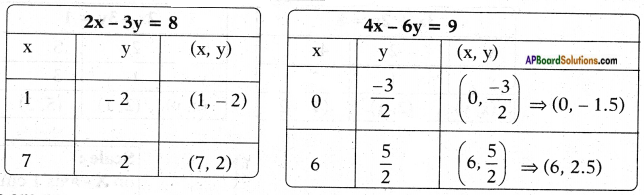Lines are parallel.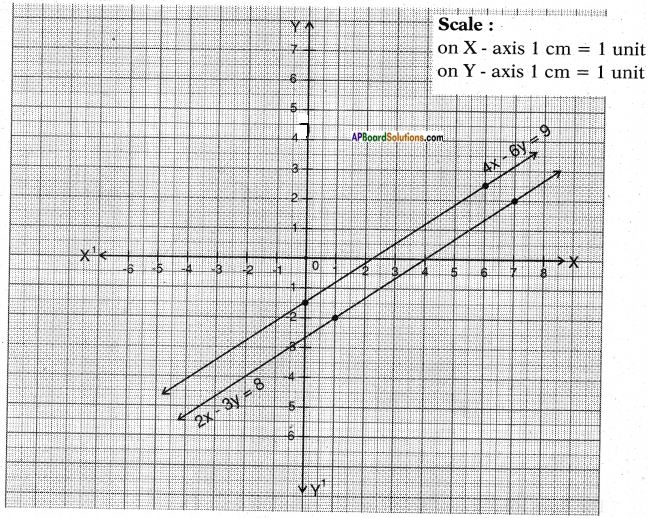The lines are parallel and no solution exists.c) $$\frac{3}{2}$$x + $$\frac{5}{3}$$y = 7
9x – 10y = 12
Given pair of equations $$\frac{3}{2}$$x + $$\frac{5}{3}$$y = 7 and 9x – 10y = 12
Now take $$\frac{3}{2}$$x + $$\frac{5}{3}$$y = 7 ⇒ $$\frac{9x+10y}{6}$$ = 7 ⇒ 9x + 10y = 42
and 9x – 10y =12
$$\frac{a_{1}}{a_{2}}$$ = $$\frac{9}{9}$$ = $$\frac{1}{1}$$;
$$\frac{b_{1}}{b_{2}}$$ = $$\frac{10}{-10}$$ = $$\frac{1}{-1}$$ and
$$\frac{c_{1}}{c_{2}}$$ = $$\frac{-42}{-12}$$ = $$\frac{7}{2}$$
Since $$\frac{a_{1}}{a_{2}}$$ ≠ $$\frac{b_{1}}{b_{2}}$$ they are intersecting lines and hence consistent pair of linear equations.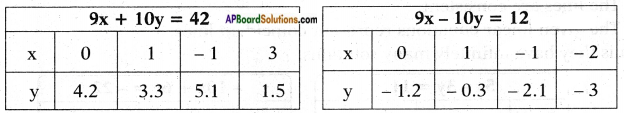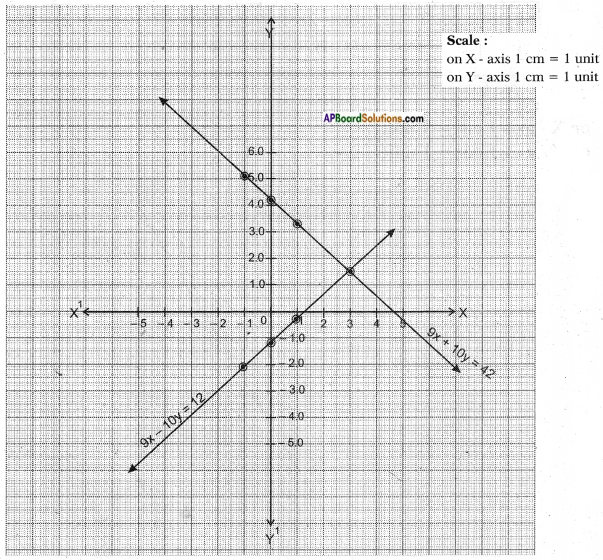Solution: The unique solution of given pair of equations is (3.1, 1.4)

d) 5x – 3y = 11
-10x + 6y = -22
Given pair of equations 5x – 3y = 11 and -10x + 6y = -22
$$\frac{a_{1}}{a_{2}}$$ = $$\frac{5}{-10}$$ = $$\frac{-1}{2}$$;
$$\frac{b_{1}}{b_{2}}$$ = $$\frac{-3}{6}$$ = $$\frac{-1}{2}$$ and
$$\frac{c_{1}}{c_{2}}$$ = $$\frac{11}{-22}$$ = $$\frac{-1}{2}$$
∴ $$\frac{a_{1}}{a_{2}}$$ = $$\frac{b_{1}}{b_{2}}$$ = $$\frac{c_{1}}{c_{2}}$$
∴ The lines are consistent.
∴ The given linear equations represent coincident lines.
Thus they have infinitely many solutions.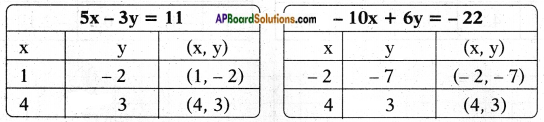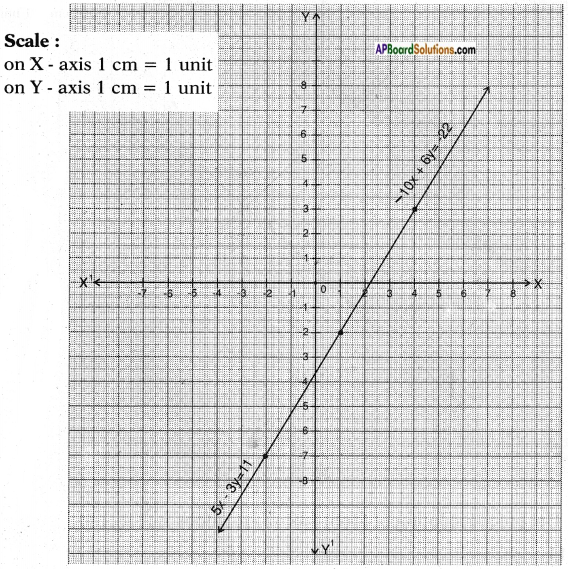e) $$\frac{4}{3}$$x + 2y = 8
2x + 3y = 12
Given pair of equations $$\frac{4}{3}$$x + 2y = 8 ⇒ $$\frac{4x+6y}{3}$$ = 8 ⇒ 4x + 6y = 24 ⇒ 2x + 3y = 12
$$\frac{a_{1}}{a_{2}}$$ = $$\frac{4}{2}$$ = 2;
$$\frac{b_{1}}{b_{2}}$$ = $$\frac{6}{3}$$ = 2;
$$\frac{c_{1}}{c_{2}}$$ = $$\frac{24}{12}$$ = 2
∴ $$\frac{a_{1}}{a_{2}}$$ = $$\frac{b_{1}}{b_{2}}$$ = $$\frac{c_{1}}{c_{2}}$$
Thus the equations are consistent.
∴ The given equations have infinitely many solutions.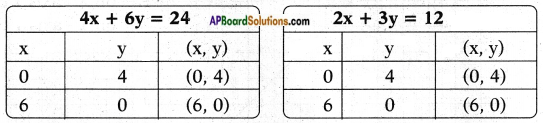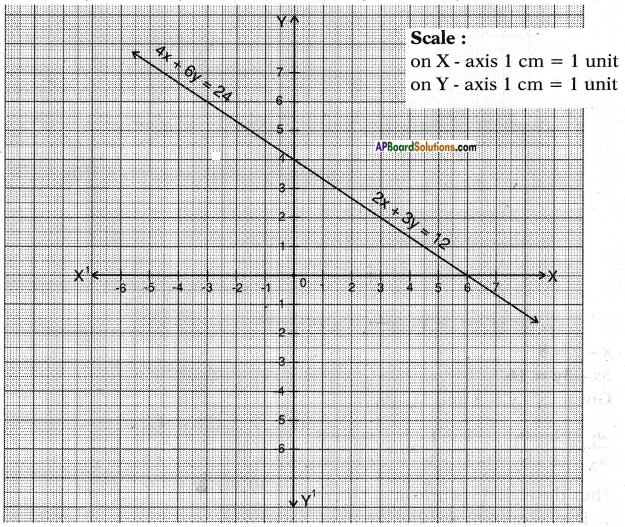f) x + y = 5
2x + 2y = 10
Given pair of equations x + y = 5 and 2x + 2y = 10
$$\frac{a_{1}}{a_{2}}$$ = $$\frac{1}{2}$$;
$$\frac{b_{1}}{b_{2}}$$ = $$\frac{1}{2}$$;
$$\frac{c_{1}}{c_{2}}$$ = $$\frac{5}{10}$$ = $$\frac{1}{2}$$
∴ $$\frac{a_{1}}{a_{2}}$$ = $$\frac{b_{1}}{b_{2}}$$ = $$\frac{c_{1}}{c_{2}}$$
Thus the equations are consistent and have infinitely many solutions.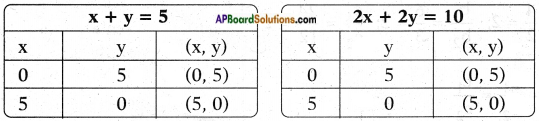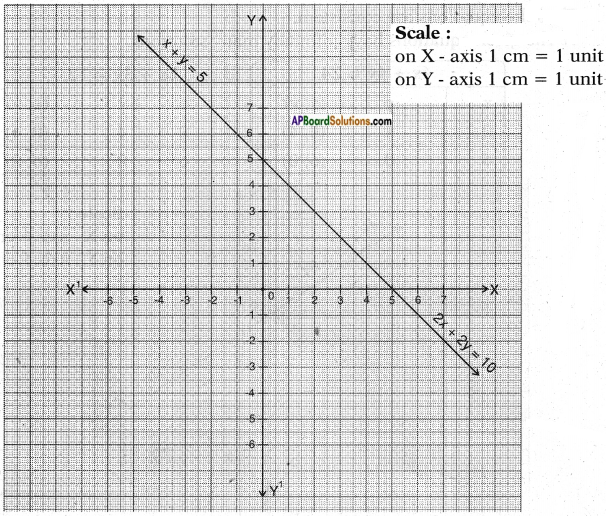g) x – y = 8
3x – 3y = 16
Given pair of equations x – y = 8 and 3x – 3y = 16
$$\frac{a_{1}}{a_{2}}$$ = $$\frac{1}{3}$$;
$$\frac{b_{1}}{b_{2}}$$ = $$\frac{-1}{-3}$$ = $$\frac{1}{3}$$ and
$$\frac{c_{1}}{c_{2}}$$ = $$\frac{8}{16}$$ = $$\frac{1}{2}$$
∴ $$\frac{a_{1}}{a_{2}}$$ = $$\frac{b_{1}}{b_{2}}$$ ≠ $$\frac{c_{1}}{c_{2}}$$
Thus the equations are inconsistent.
∴ They represent parallel lines and have no solution.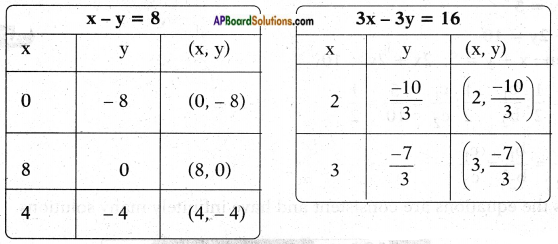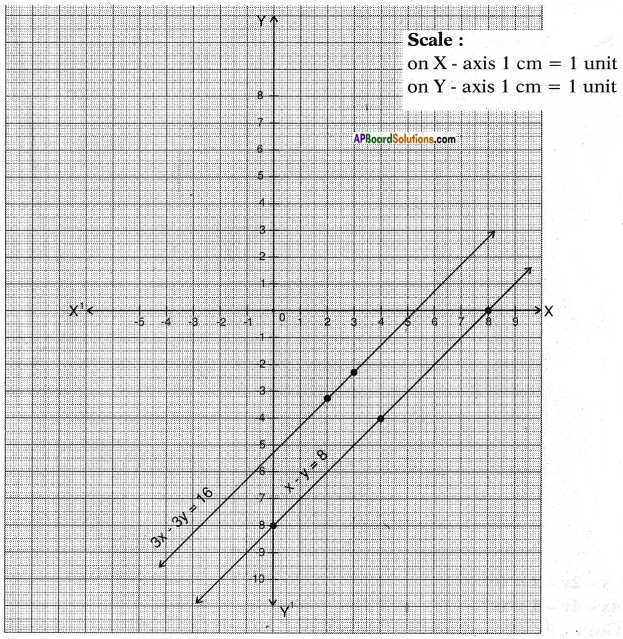h) 2x + y – 6 = 0 and 4x – 2y – 4 = 0
Given pair of equations 2x + y – 6 = 0 and 4x – 2y – 4 = 0
$$\frac{a_{1}}{a_{2}}$$ = $$\frac{2}{4}$$ = $$\frac{1}{2}$$;
$$\frac{b_{1}}{b_{2}}$$ = $$\frac{1}{-2}$$ = $$\frac{-1}{2}$$;
$$\frac{c_{1}}{c_{2}}$$ = $$\frac{-6}{-4}$$ = $$\frac{3}{2}$$
∴ $$\frac{a_{1}}{a_{2}}$$ ≠ $$\frac{b_{1}}{b_{2}}$$
The equations are consistent.
∴ They intersect at one point giving only one solution.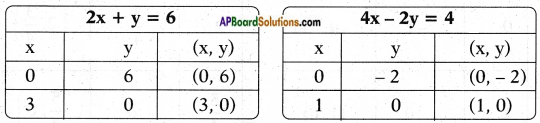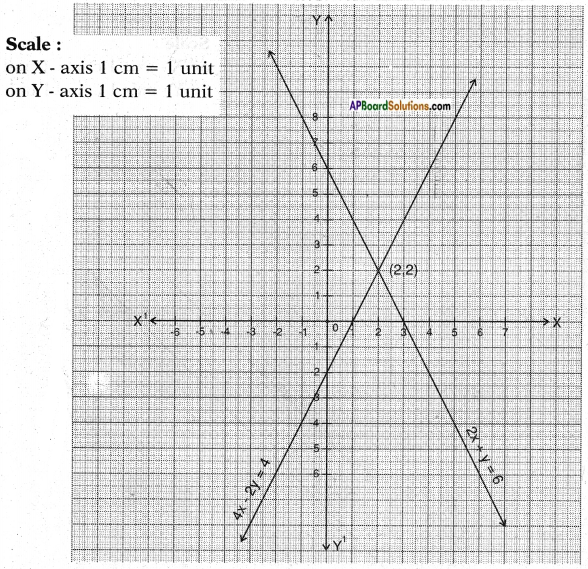The solution is x = 2 and y = 2i) 2x – 2y – 2 = 0 and 4x – 4y – 5 = 0
Given pair of equations 2x – 2y – 2 = 0 and 4x – 4y – 5 = 0
$$\frac{a_{1}}{a_{2}}$$ = $$\frac{2}{4}$$ = $$\frac{1}{2}$$;
$$\frac{b_{1}}{b_{2}}$$ = $$\frac{-2}{-4}$$ = $$\frac{1}{2}$$;
$$\frac{c_{1}}{c_{2}}$$ = $$\frac{-2}{-5}$$ = $$\frac{2}{5}$$
∴ $$\frac{a_{1}}{a_{2}}$$ = $$\frac{b_{1}}{b_{2}}$$ ≠ $$\frac{c_{1}}{c_{2}}$$
Thus the equations are inconsistent.
∴ They represent parallel lines and have no solution.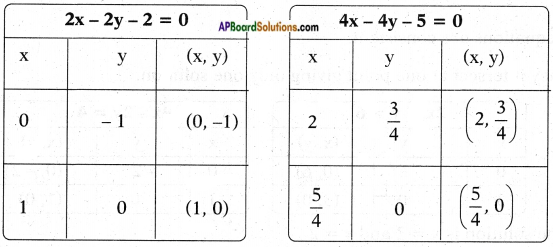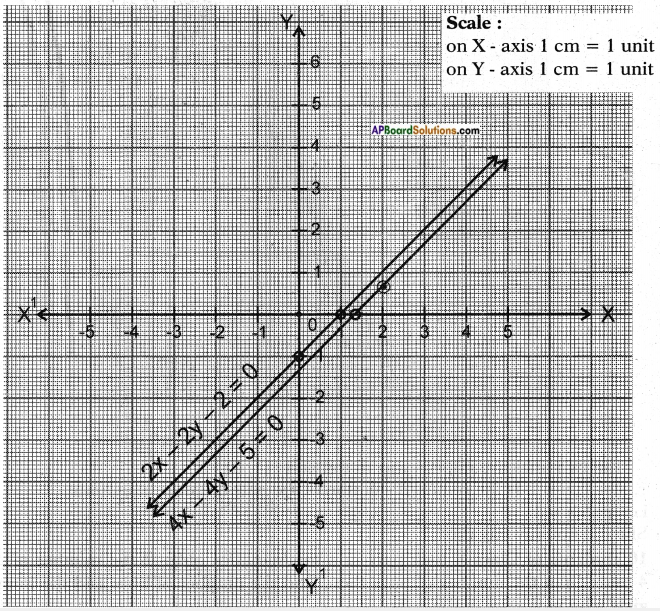Question 3.
Neha went to a ‘sale’ to purchase some pants and skirts. When her friend asked her how many of each she had bought, she answered “The number of skirts are two less than twice the number of pants purchased. Also the number of skirts is four less than four times the number of pants purchased.”
Help her friend to find how many pants and skirts Neha bought.
Let the number of pants = x and the number of skirts = y
By problem y = 2x – 2 ⇒ 2x – y = 2
y = 4x – 4 ⇒ 4x – y = 4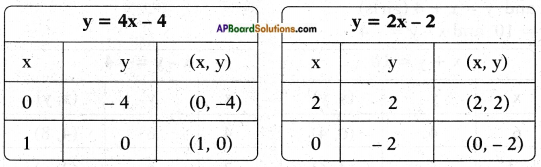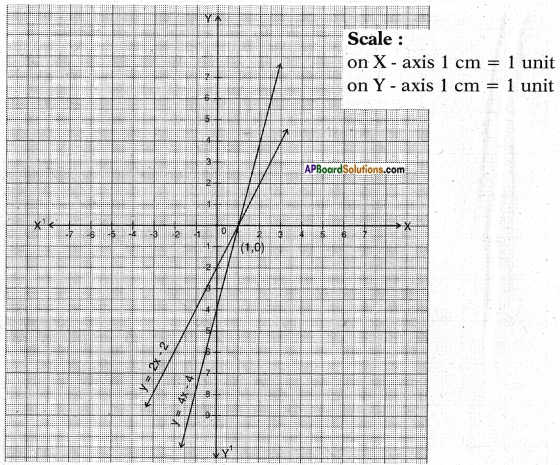The two lines are intersecting at the point (1,0)
∴ x = 1; y = 0 is the required solution of the pair of linear equations.
i.e., pants =1
She did not buy any skirt.Question 4.
10 students of Class-X took part in a mathematics quiz. If the number of girls is 4 more than the number of boys then, find the number of boys and the number of girls who took part in the quiz.
Let the number of boys be x.
Then the number of girls = x + 4
By problem, x + x + 4 = 10
∴ 2x + 4 = 10
2x = 10-4
x = $$\frac{6}{2}$$ = 3
∴ Boys = 3 Girls = 3 + 4 = 7 (or)
Boys = x, Girls = y
By problem x + y = 10 (total)
and y = x + 4 (girls)
⇒ x + y = 10 and x – y = – 4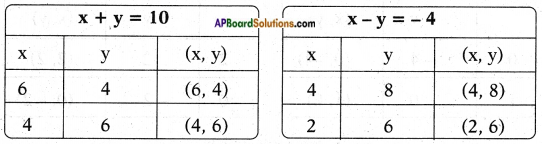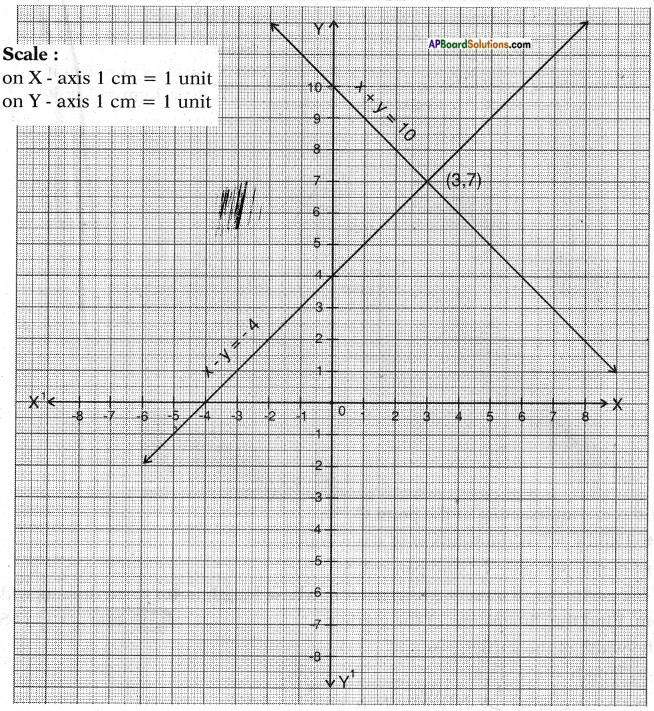∴ Number of boys = 3 and the number of girls = 7

Question 5.
5 pencils and 7 pens together cost Rs. 50 whereas 7 pencils and 5 pens together cost Rs. 46. Find the cost of one pencil and that of one pen.
Let the cost of each pencil be Rs. x
and the cost of each pen be Rs. y.
By problem 5x + 7y = 50
7x + 5y = 46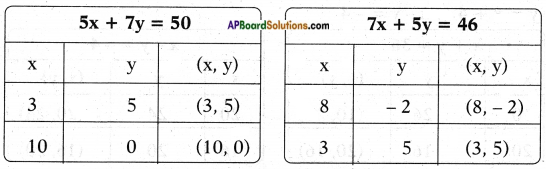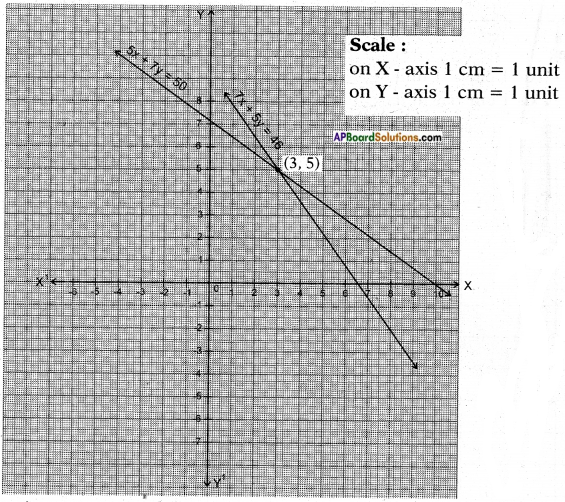The lines are intersecting at the point (3, 5).
x = 3 and y = 5 is the solution of given equations.
∴ Cost of one pencil = Rs. 3 and pen = Rs. 5Question 6.
Half the perimeter of a rectangular garden, whose length is 4 m more than its width is 36 m. Find the dimensions of the garden.
Let the width of the garden = x cm
then its length = x + 4 cm
Half the perimeter = $$\frac{1}{2}$$ × 2(7+ b) = l + b
By problem, x + x + 4 = 36
2x + 4 = 36
2x = 36 – 4 = 32
∴ x = 16 and x + 4 = 16 + 4 = 20
i.e., length = 20 cm and breadth = 16 cm.
(or)
Let the breadth be x and length = y
then x + y = 36 ⇒ x + y = 36
y = x + 4 ⇒ x – y = -4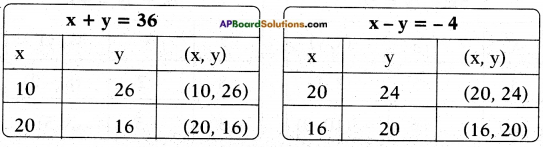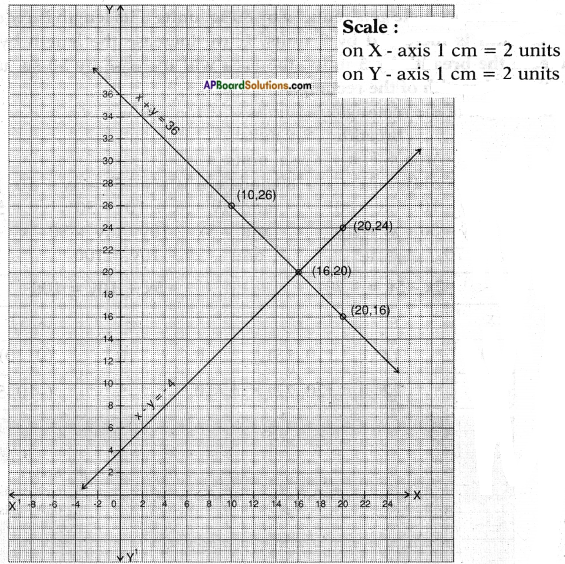The two lines intersect at the point (16, 20)
i.e., length = 20 cm and the breadth = 16 cm.

Question 7.
We have a linear equation 2x + 3y – 8 = 0. Write another linear equation in two variables such that the geometrical representation of the pair so formed is intersect¬ing lines. Now, write two more linear equations so that one forms a pair of parallel lines and the second forms coincident line with the given equation.
i) Given: 2x + 3y – 8 = 0
The lines are intersecting lines.
Let the other linear equation be ax + by + c = 0
∴ $$\frac{a_{1}}{a_{2}}$$ ≠ $$\frac{b_{1}}{b_{2}}$$; we have to choose appropriate values satisfying the condition above.
Thus the other equation may be 3x + 5y – 6 =0

ii) Parallel line $$\frac{a_{1}}{a_{2}}$$ = $$\frac{b_{1}}{b_{2}}$$ ≠ $$\frac{c_{1}}{c_{2}}$$
⇒ 2x + 3y – 8 = 0
4x + 6y – 10 = 0

iii) Coincident lines $$\frac{a_{1}}{a_{2}}$$ = $$\frac{b_{1}}{b_{2}}$$ = $$\frac{c_{1}}{c_{2}}$$
⇒ 2x + 3y – 8 = 0 ⇒ 8x + 12y – 32 = 0Question 8.
The area of a rectangle gets reduced by 80 sq. units if its length is reduced by 5 units and breadth is increased by 2 units. If we increase the length by 10 units and decrease the breadth by 5 units, the area will increase by 50 sq. units. Find the length and breadth of the rectangle.
Let the length of the rectangle = x units
breadth = y units Area = l . b = xy sq. units
By problem, (x – 5) (y + 2) = xy – 80 and          (x + 10) (y – 5) = xy + 50
⇒ xy + 2x – 5y – 10 = xy – 80 and                    xy – 5x + 10y – 50 = xy + 50
⇒ 2x – 5y = xy – 80 – xy + 10 and                   -5x + 10y = xy + 50 – xy + 50
⇒ 2x – 5y = – 70 and                                       -5x + 10y = 100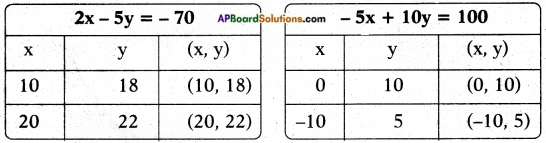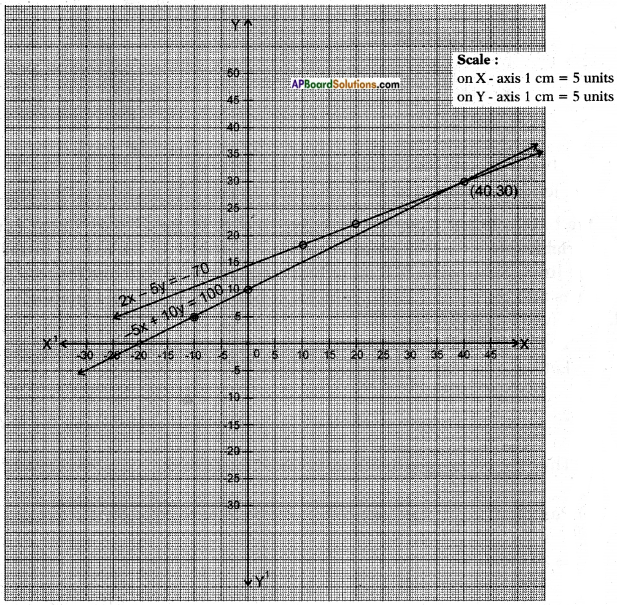The two lines intersect at the point (40, 30)
∴ The solution is x = 40 and y = 30
i.e., length = 40 units; breadth = 30 units.Question 9.
In X class, if three students sit on each bench, one student will be left. If four students sit on each bench, one bench will be left. Find the number of students and the number of benches in that class.